# Matrix Operations in Image Processing

Recoloring, the process of changing image colors, is a good example of color transformation. Recoloring includes changing colors, intensity, contrast, and brightness of an image. It can all be done via the ImageAttributes class and its methods.

The color matrix can be applied to an image via the SetColorMatrix method of the ImageAttributes class. The ImageAttributes object is used as a parameter when we call DrawImage.

10.8.1 Translating Colors

Translating colors increases or decreases color intensities by a set amount (not by multiplying them). Each color component (red, green, and blue) has 255 different intensity levels ranging from 0 to 255. For example, assume that the current intensity level for the red component of a color is 100. Changing its intensity level to 150 would imply translating by 50.

In a color matrix representation, the intensity varies from 0 to 1. The last row's first four elements represent the translation of red, green, blue, and alpha components of a color, as shown in Figure 10.22. Hence, adding a value to these elements will transform a color. For example, the t1, t2, t3, and t4 values in the following color matrix represent the red, green, blue, and alpha component translations, respectively:

```
```
```Color Matrix = {
{1, 0, 0, 0, 0},
{0, 1, 0, 0, 0},
{0, 0, 1, 0, 0},
{0, 0, 0, 1, 0},
{t1, t2, t3, t4, 1}};
```
```
```

Listing 10.18 uses a ColorMatrix object to translate colors. We change the current intensity of the red component to 0.90. First we create a Graphics object using the CreateGraphics method, and we create a Bitmap object from a file. Next we create an array of ColorMatrix elements and create a ColorMatrix object from this array. Then we create an ImageAttributes object and set the color matrix using SetColorMatrix, which takes the ColorMatrix object as its first parameter. After all that, we draw two images. The first image has no effects; the second image shows the result of our color matrix transformation. Finally, we dispose of the objects.

Listing 10.18 Using ColorMatrix to translate colors

```private void TranslationMenu_Click(object sender,
System.EventArgs e)
{
// Create a Graphics object
Graphics g = this.CreateGraphics();
g.Clear(this.BackColor);
// Create a Bitmap object
Bitmap curBitmap = new Bitmap("roses.jpg");
// Color matrix elements
float[][] ptsArray =
{
new float[] {1, 0, 0, 0, 0},
new float[] {0, 1, 0, 0, 0},
new float[] {0, 0, 1, 0, 0},
new float[] {0, 0, 0, 1, 0},
new float[] {.90f, .0f, .0f, .0f, 1}
};
// Create a ColorMatrix object
ColorMatrix clrMatrix = new ColorMatrix(ptsArray);
// Create image attributes
ImageAttributes imgAttribs = new ImageAttributes();
// Set color matrix
imgAttribs.SetColorMatrix(clrMatrix,
ColorMatrixFlag.Default,
// Draw image with no effects
g.DrawImage(curBitmap, 0, 0, 200, 200);
// Draw image with image attributes
g.DrawImage(curBitmap,
new Rectangle(205, 0, 200, 200),
0, 0, curBitmap.Width, curBitmap.Height,
GraphicsUnit.Pixel, imgAttribs) ;
// Dispose of objects
curBitmap.Dispose();
g.Dispose();
}
```

Figure 10.24 shows the output from Listing 10.18. The original image is on the left; on the right we have the results of our color translation. If you change the values of other components (red, blue, and alpha) in the last row of the color matrix, you'll see different results.

Figure 10.24. Translating colors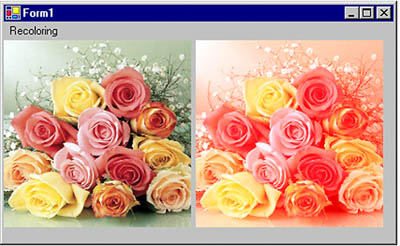10.8.2 Scaling Colors

Scaling color involves multiplying a color component value by a scaling factor. For example, the t1, t2, t3, and t4 values in the following color matrix represent the red, green, blue, and alpha components, respectively. If we change the value of M to 0.5, the transformation operation will multiply the green component by 0.5, cutting its intensity by half.

```
```
```Color Matrix = {
{t1, 0, 0, 0, 0},
{0, t2, 0, 0, 0},
{0, 0, t3, 0, 0},
{0, 0, 0, t4, 0},
{0, 0, 0, 0, 1}};
```
```
```

Listing 10.19 uses the ColorMatrix object to scale image colors.

Listing 10.19 Scaling colors

```private void ScalingMenu_Click(object sender,
System.EventArgs e)
{
// Create a Graphics object
Graphics g = this.CreateGraphics();
g.Clear(this.BackColor);
// Create a Bitmap object
Bitmap curBitmap = new Bitmap("roses.jpg");
// Color matrix elements
float[][] ptsArray =
{
new float[] {1, 0, 0, 0, 0},
new float[] {0, 0.8f, 0, 0, 0},
new float[] {0, 0, 0.5f, 0, 0},
new float[] {0, 0, 0, 0.5f, 0},
new float[] {0, 0, 0, 0, 1}
};
// Create a ColorMatrix object
ColorMatrix clrMatrix = new ColorMatrix(ptsArray);
// Create image attributes
ImageAttributes imgAttribs = new ImageAttributes();
// Set color matrix
imgAttribs.SetColorMatrix(clrMatrix,
ColorMatrixFlag.Default,
// Draw image with no effects
g.DrawImage(curBitmap, 0, 0, 200, 200);
// Draw image with image attributes
g.DrawImage(curBitmap,
new Rectangle(205, 0, 200, 200),
0, 0, curBitmap.Width, curBitmap.Height,
GraphicsUnit.Pixel, imgAttribs) ;
// Dispose of objects
curBitmap.Dispose();
g.Dispose();
}
```

Figure 10.25 shows the output from Listing 10.19. The original image is on the left; on the right is the image after color scaling. If you change the values of t1, t2, t3, and t4, you will see different results.

Figure 10.25. Scaling colors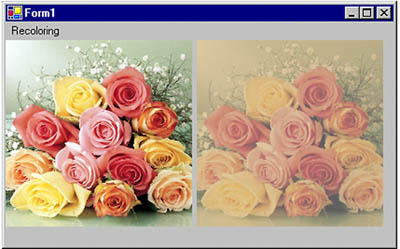10.8.3 Shearing Colors

Earlier in this chapter we discussed image shearing. It can be thought of as anchoring one corner of a rectangular region and stretching the opposite corner horizontally, vertically, or in both directions. Shearing colors is the same process, but here the object is the color instead of the image.

Color shearing increases or decreases a color component by an amount proportional to another color component. For example, consider the transformation in which the red component is increased by one half the value of the blue component. Under such a transformation, the color (0.2, 0.5, 1) would become (0.7, 0.5, 1). The new red component is 0.2 + (0.5)(1) = 0.7. The following color matrix is used to shear image colors.

```
```
```float[][] ptsArray = {
new float[] {1, 0, 0, 0, 0},
new float[] {0, 1, 0, 0, 0},
new float[] {.50f, 0, 1, 0, 0},
new float[] {0, 0, 0, 1, 0},
new float[] {0, 0, 0, 0, 1}};
ColorMatrix clrMatrix = new ColorMatrix(ptsArray);
```
```
```

If we substitute this color matrix into Listing 10.19, the output will look like Figure 10.26.

Figure 10.26. Shearing colors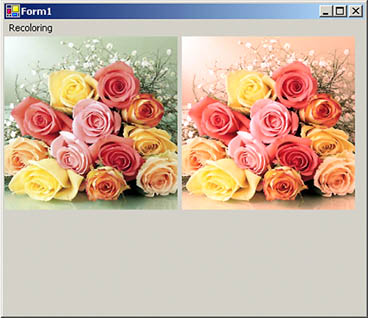10.8.4 Rotating Colors

As explained earlier, color in GDI+ has four components: red, green, blue, and alpha. Rotating all four components in a four-dimensional space is hard to visualize. However, such rotation can be visualized in a three-dimensional space. To do this, we drop the alpha component from the color structure and assume that there are only three colorsred, green, and blueas shown in Figure 10.27. The three colorsred, green, and blueare perpendicular to each other, so the angle between any two primary colors is 90 degrees.

Figure 10.27. RGB rotation space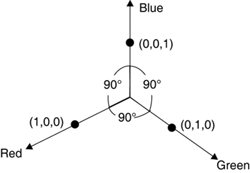Suppose that the red, green, and blue colors are represented by points (1, 0, 0), (0, 1, 0), and (0, 0, 1), respectively. If we rotate a color with a green component of 1, and red and blue components of 0 each, by 90 degrees, the new color will have a red component of 1, and green and blue components of 0 each. If we rotate the color less than 90 degrees, the new color will be located somewhere between green and red.

Figure 10.28 shows how to initialize a color matrix to perform rotations about each of the three components: red, green, and blue.

Figure 10.28. RGB initialization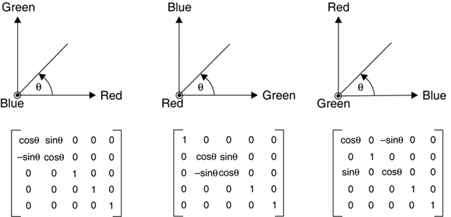Listing 10.20 rotates the colors by 45 degrees from the red component.

Listing 10.20 Rotating colors

```private void RotationMenu_Click(object sender,
System.EventArgs e)
{
float degrees = 45.0f;
double r = degrees*System.Math.PI/180;
// Create a Graphics object
Graphics g = this.CreateGraphics();
g.Clear(this.BackColor);
// Create a Bitmap object from a file
Bitmap curBitmap = new Bitmap("roses.jpg");
// Color matrix elements
float[][] ptsArray =
{
new float[] {(float)System.Math.Cos(r),
(float)System.Math.Sin(r),
0, 0, 0},
new float[] {(float)-System.Math.Sin(r),
(float)-System.Math.Cos(r),
0, 0, 0},
new float[] {.50f, 0, 1, 0, 0},
new float[] {0, 0, 0, 1, 0},
new float[] {0, 0, 0, 0, 1}
};
// Create a ColorMatrix object
ColorMatrix clrMatrix = new ColorMatrix(ptsArray);
// Create image attributes
ImageAttributes imgAttribs = new ImageAttributes();
// Set ColorMatrix to ImageAttributes
imgAttribs.SetColorMatrix(clrMatrix,
ColorMatrixFlag.Default,
// Draw image with no effects
g.DrawImage(curBitmap, 0, 0, 200, 200);
// Draw image with image attributes
g.DrawImage(curBitmap,
new Rectangle(205, 0, 200, 200),
0, 0, curBitmap.Width, curBitmap.Height,
GraphicsUnit.Pixel, imgAttribs) ;
// Dispose of objects
curBitmap.Dispose();
g.Dispose();
}
```

Figure 10.29 slows the output from Listing 10.20. On the left is the original image; on the right is the image after color rotation.

Figure 10.29. Rotating colors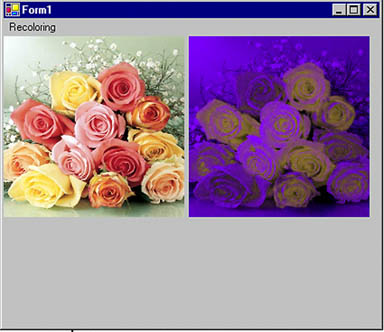GDI+ Programming with C#
ISBN: 073561265X
EAN: N/A
Year: 2003
Pages: 145